Скачать презентацию Chapter 7 Product Variety and Quality under monopoly

eab32eda709b26ed884b91d9ab895ea6.ppt

• Количество слайдов: 44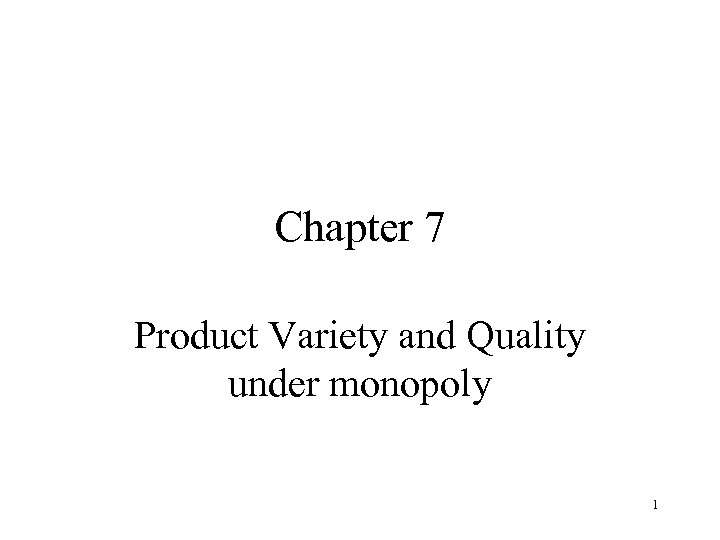Chapter 7 Product Variety and Quality under monopoly 1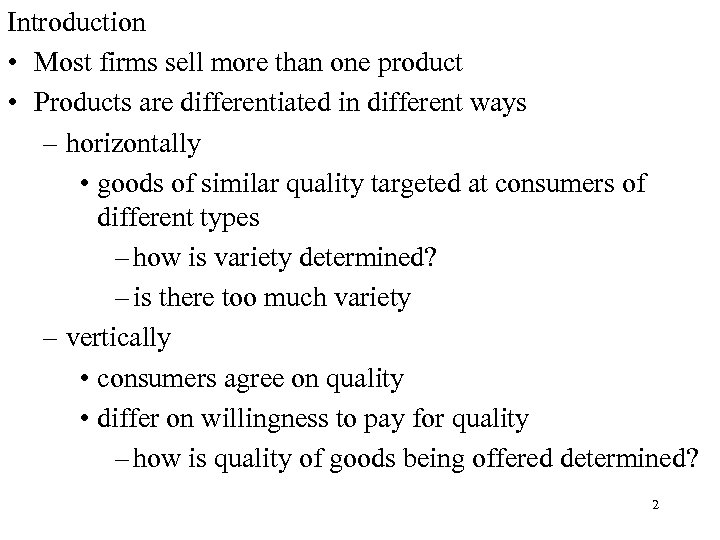Introduction • Most firms sell more than one product • Products are differentiated in different ways – horizontally • goods of similar quality targeted at consumers of different types – how is variety determined? – is there too much variety – vertically • consumers agree on quality • differ on willingness to pay for quality – how is quality of goods being offered determined? 2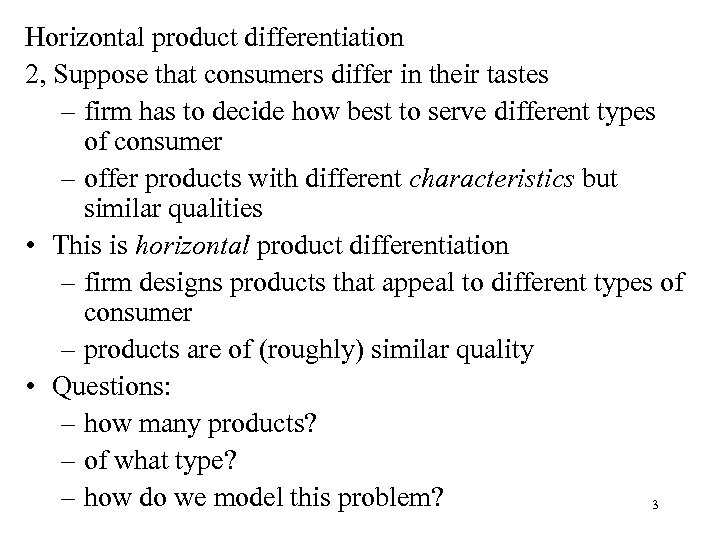Horizontal product differentiation 2, Suppose that consumers differ in their tastes – firm has to decide how best to serve different types of consumer – offer products with different characteristics but similar qualities • This is horizontal product differentiation – firm designs products that appeal to different types of consumer – products are of (roughly) similar quality • Questions: – how many products? – of what type? – how do we model this problem? 3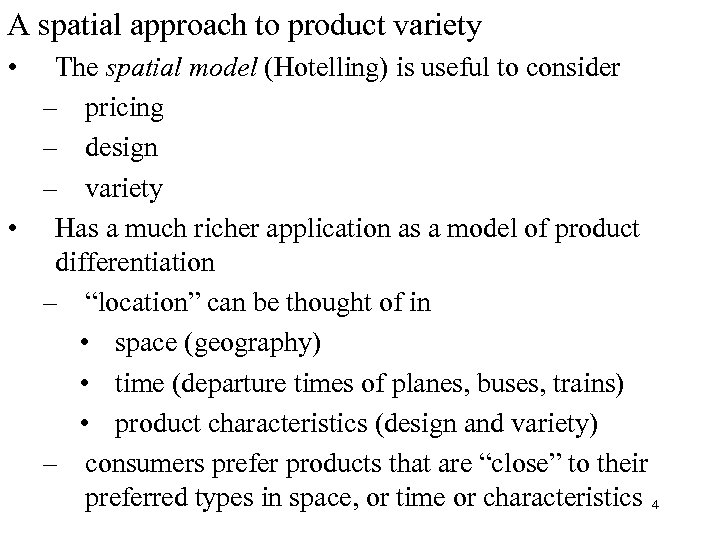A spatial approach to product variety • The spatial model (Hotelling) is useful to consider – pricing – design – variety • Has a much richer application as a model of product differentiation – “location” can be thought of in • space (geography) • time (departure times of planes, buses, trains) • product characteristics (design and variety) – consumers prefer products that are “close” to their preferred types in space, or time or characteristics 4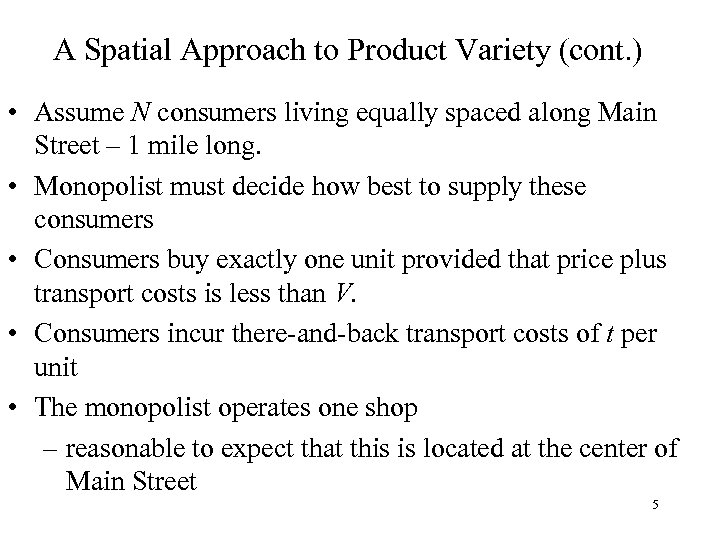A Spatial Approach to Product Variety (cont. ) • Assume N consumers living equally spaced along Main Street – 1 mile long. • Monopolist must decide how best to supply these consumers • Consumers buy exactly one unit provided that price plus transport costs is less than V. • Consumers incur there-and-back transport costs of t per unit • The monopolist operates one shop – reasonable to expect that this is located at the center of Main Street 5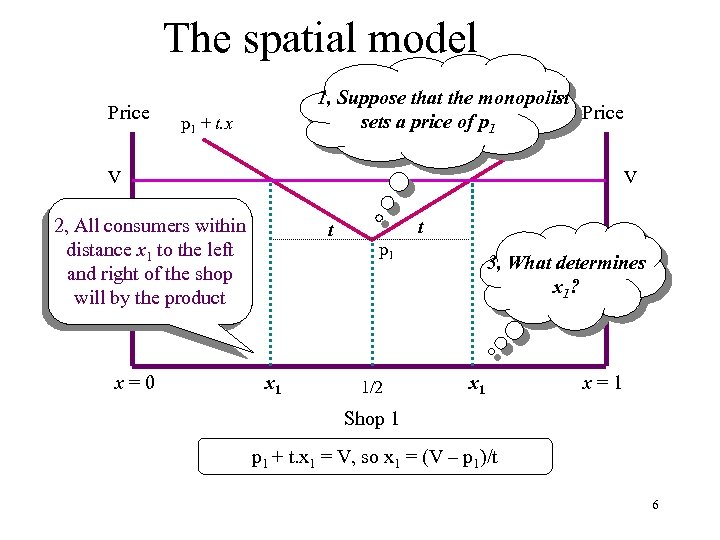The spatial model Price 1, Suppose that the monopolist Price sets a price of p 1 p 1 + t. x V V 2, All consumers within distance x 1 to the left and right of the shop will by the product x=0 t x 1 t p 1 1/2 3, What determines x 1? x 1 x=1 Shop 1 + t. x 1 = V, so x 1 = (V – p 1)/t 6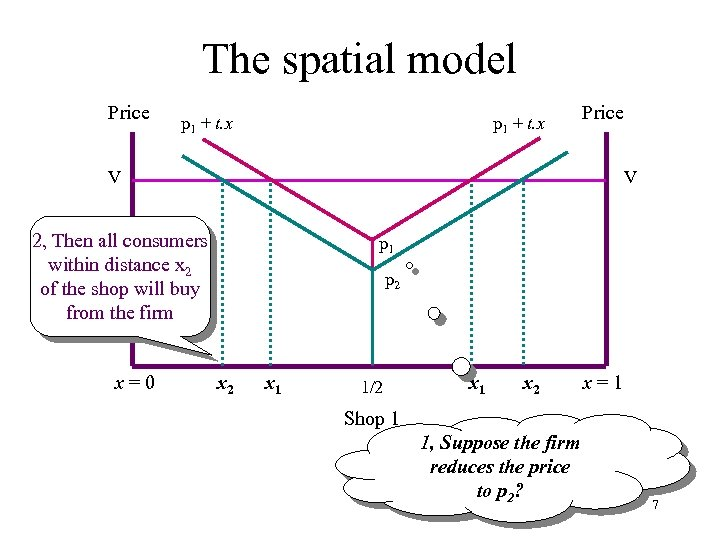The spatial model Price p 1 + t. x Price V V 2, Then all consumers within distance x 2 of the shop will buy from the firm x=0 p 1 p 2 x 1 1/2 x 1 x 2 x=1 Shop 1 1, Suppose the firm reduces the price to p 2? 7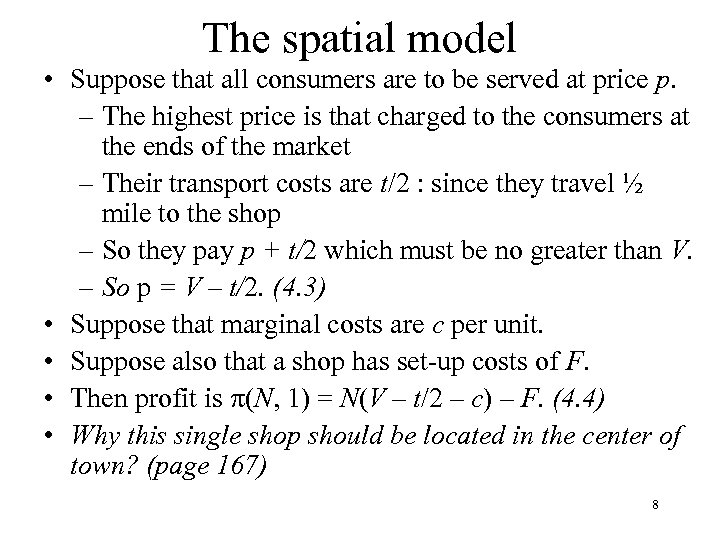The spatial model • Suppose that all consumers are to be served at price p. – The highest price is that charged to the consumers at the ends of the market – Their transport costs are t/2 : since they travel ½ mile to the shop – So they pay p + t/2 which must be no greater than V. – So p = V – t/2. (4. 3) • Suppose that marginal costs are c per unit. • Suppose also that a shop has set-up costs of F. • Then profit is p(N, 1) = N(V – t/2 – c) – F. (4. 4) • Why this single shop should be located in the center of town? (page 167) 8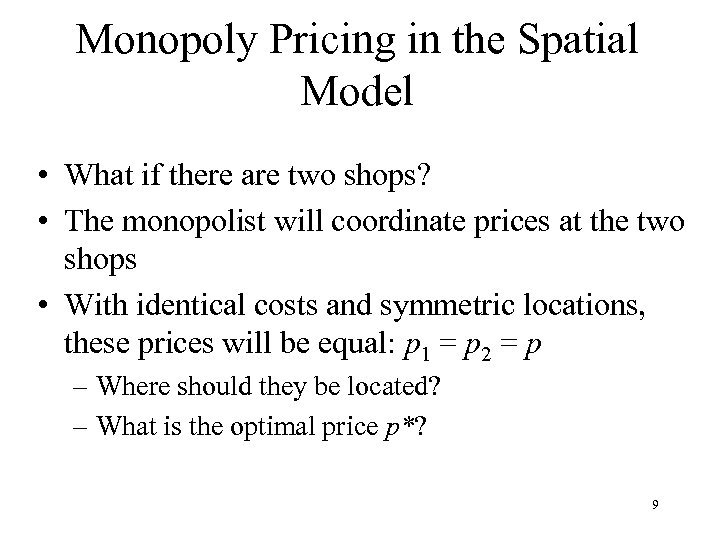Monopoly Pricing in the Spatial Model • What if there are two shops? • The monopolist will coordinate prices at the two shops • With identical costs and symmetric locations, these prices will be equal: p 1 = p 2 = p – Where should they be located? – What is the optimal price p*? 9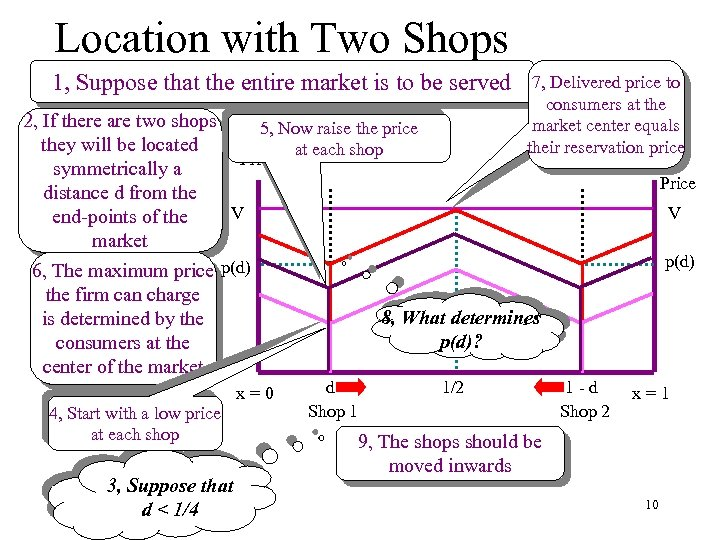Location with Two Shops 1, Suppose that the entire market is to be served 7, Delivered price to consumers at the market center equals their reservation price 2, If there are two shops 5, Now raise the price they will be located at each shop Price symmetrically a distance d from the V end-points of the market 6, The maximum price p(d) the firm can charge 8, What determines is determined by the p(d)? consumers at the center of the market 4, Start with a low price at each shop 3, Suppose that d < 1/4 x=0 d Shop 1 1/2 Price V p(d) 1 -d Shop 2 x=1 9, The shops should be moved inwards 10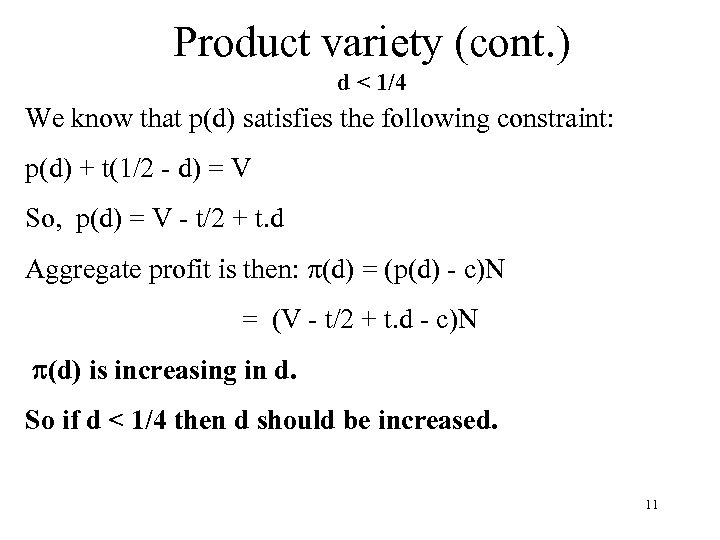Product variety (cont. ) d < 1/4 We know that p(d) satisfies the following constraint: p(d) + t(1/2 - d) = V So, p(d) = V - t/2 + t. d Aggregate profit is then: p(d) = (p(d) - c)N = (V - t/2 + t. d - c)N p(d) is increasing in d. So if d < 1/4 then d should be increased. 11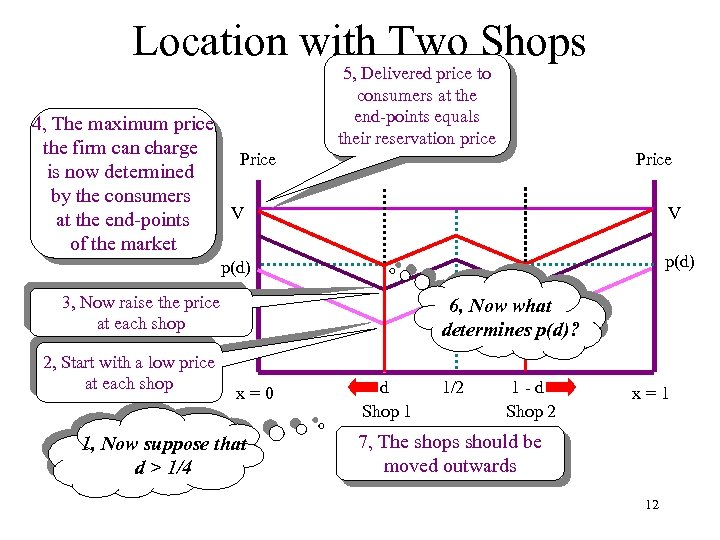Location with Two Shops 4, The maximum price the firm can charge Price is now determined by the consumers V at the end-points of the market 5, Delivered price to consumers at the end-points equals their reservation price Price V p(d) 3, Now raise the price at each shop 2, Start with a low price at each shop 6, Now what determines p(d)? x=0 1, Now suppose that d > 1/4 d Shop 1 1/2 1 -d Shop 2 x=1 7, The shops should be moved outwards 12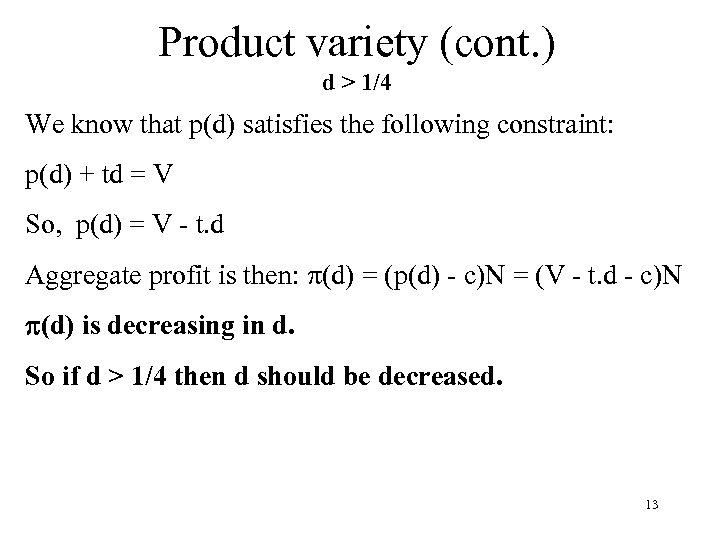Product variety (cont. ) d > 1/4 We know that p(d) satisfies the following constraint: p(d) + td = V So, p(d) = V - t. d Aggregate profit is then: p(d) = (p(d) - c)N = (V - t. d - c)N p(d) is decreasing in d. So if d > 1/4 then d should be decreased. 13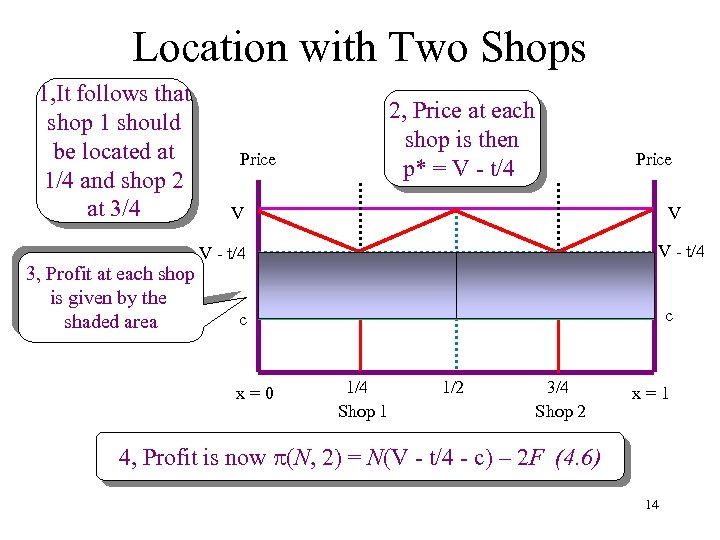Location with Two Shops 1, It follows that shop 1 should be located at 1/4 and shop 2 at 3/4 2, Price at each shop is then p* = V - t/4 Price V V V - t/4 3, Profit at each shop is given by the shaded area c c x=0 1/4 Shop 1 1/2 3/4 Shop 2 x=1 4, Profit is now p(N, 2) = N(V - t/4 - c) – 2 F (4. 6) 14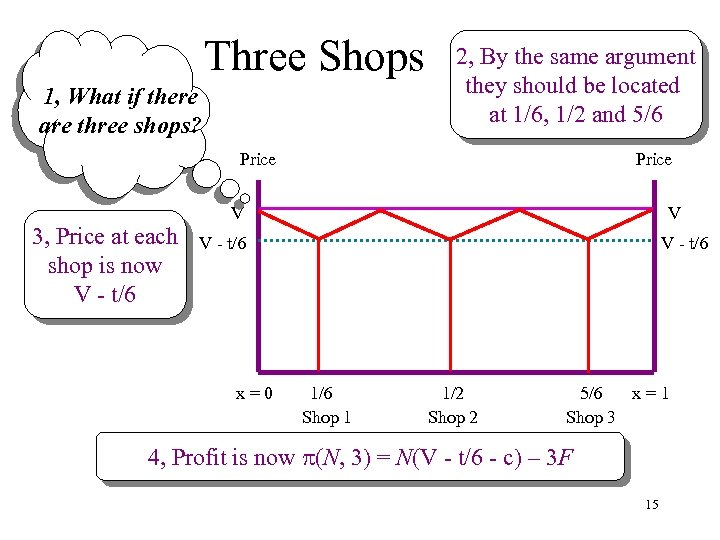Three Shops 1, What if there are three shops? 2, By the same argument they should be located at 1/6, 1/2 and 5/6 Price 3, Price at each shop is now V - t/6 Price V V V - t/6 x=0 V - t/6 1/6 Shop 1 1/2 Shop 2 5/6 x=1 Shop 3 4, Profit is now p(N, 3) = N(V - t/6 - c) – 3 F 15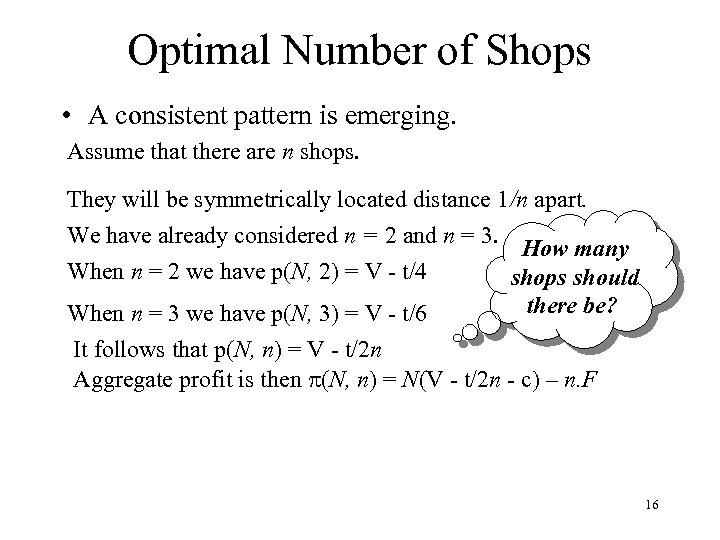Optimal Number of Shops • A consistent pattern is emerging. Assume that there are n shops. They will be symmetrically located distance 1/n apart. We have already considered n = 2 and n = 3. How many When n = 2 we have p(N, 2) = V - t/4 shops should there be? When n = 3 we have p(N, 3) = V - t/6 It follows that p(N, n) = V - t/2 n Aggregate profit is then p(N, n) = N(V - t/2 n - c) – n. F 16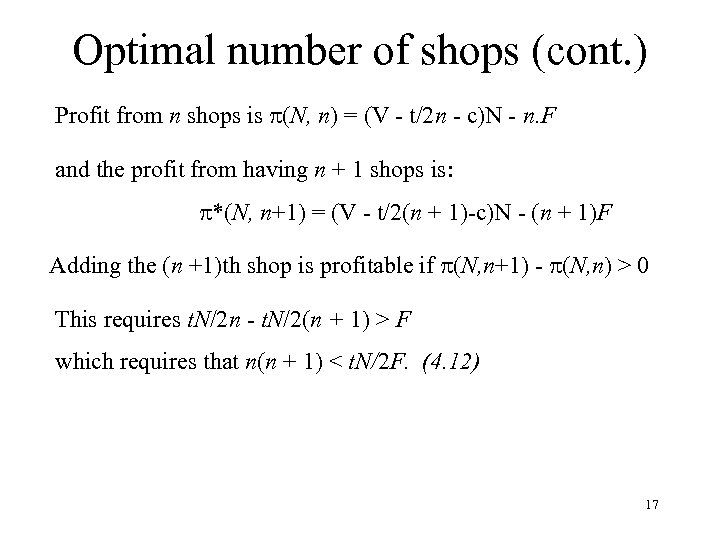Optimal number of shops (cont. ) Profit from n shops is p(N, n) = (V - t/2 n - c)N - n. F and the profit from having n + 1 shops is: p*(N, n+1) = (V - t/2(n + 1)-c)N - (n + 1)F Adding the (n +1)th shop is profitable if p(N, n+1) - p(N, n) > 0 This requires t. N/2 n - t. N/2(n + 1) > F which requires that n(n + 1) < t. N/2 F. (4. 12) 17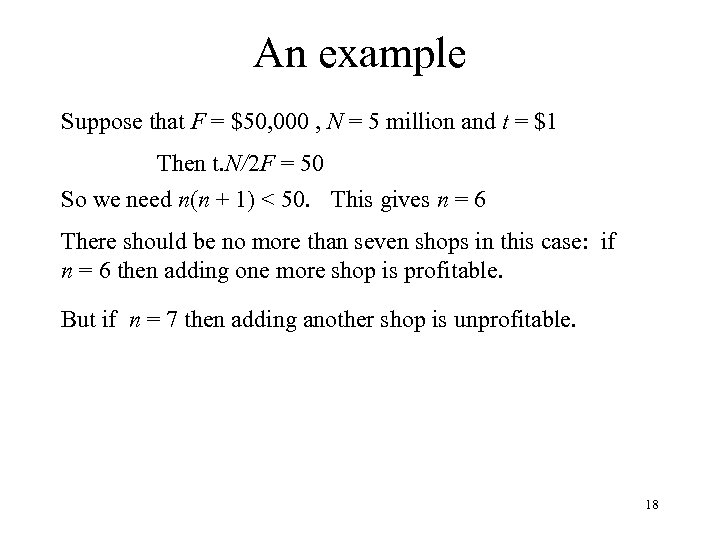An example Suppose that F = \$50, 000 , N = 5 million and t = \$1 Then t. N/2 F = 50 So we need n(n + 1) < 50. This gives n = 6 There should be no more than seven shops in this case: if n = 6 then adding one more shop is profitable. But if n = 7 then adding another shop is unprofitable. 18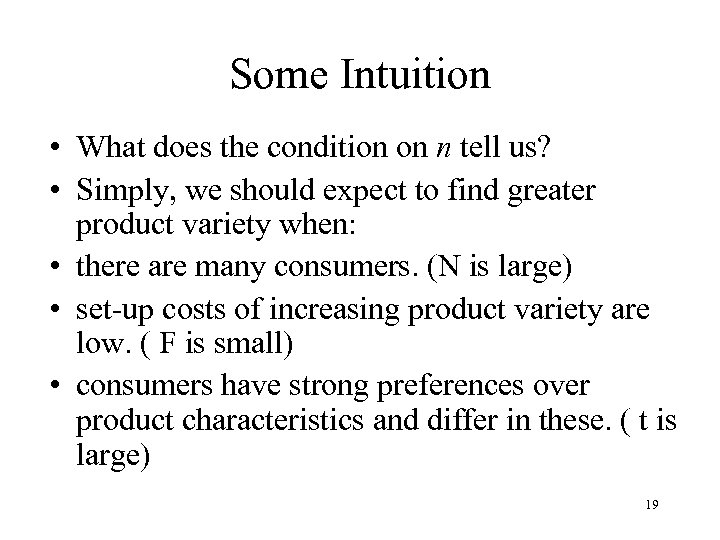Some Intuition • What does the condition on n tell us? • Simply, we should expect to find greater product variety when: • there are many consumers. (N is large) • set-up costs of increasing product variety are low. ( F is small) • consumers have strong preferences over product characteristics and differ in these. ( t is large) 19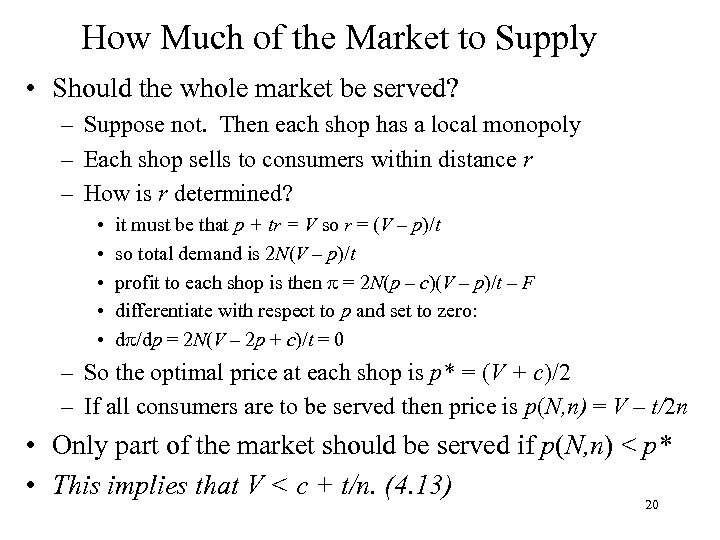How Much of the Market to Supply • Should the whole market be served? – Suppose not. Then each shop has a local monopoly – Each shop sells to consumers within distance r – How is r determined? • • • it must be that p + tr = V so r = (V – p)/t so total demand is 2 N(V – p)/t profit to each shop is then p = 2 N(p – c)(V – p)/t – F differentiate with respect to p and set to zero: dp/dp = 2 N(V – 2 p + c)/t = 0 – So the optimal price at each shop is p* = (V + c)/2 – If all consumers are to be served then price is p(N, n) = V – t/2 n • Only part of the market should be served if p(N, n) < p* • This implies that V < c + t/n. (4. 13) 20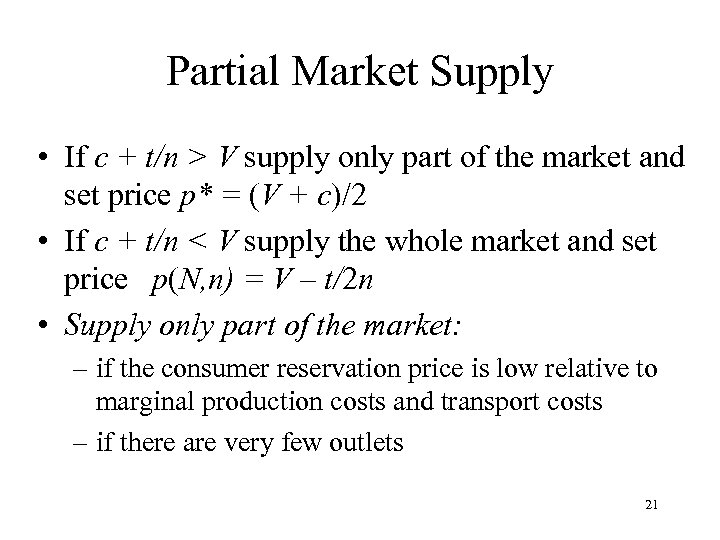Partial Market Supply • If c + t/n > V supply only part of the market and set price p* = (V + c)/2 • If c + t/n < V supply the whole market and set price p(N, n) = V – t/2 n • Supply only part of the market: – if the consumer reservation price is low relative to marginal production costs and transport costs – if there are very few outlets 21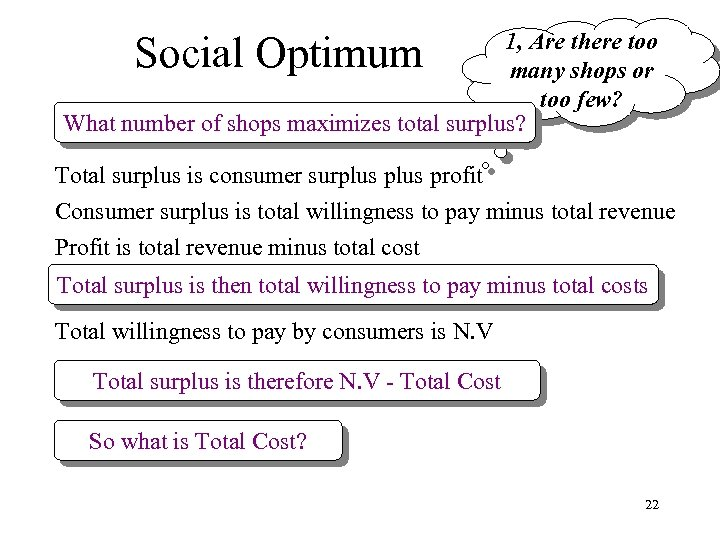Social Optimum 1, Are there too many shops or too few? What number of shops maximizes total surplus? Total surplus is consumer surplus profit Consumer surplus is total willingness to pay minus total revenue Profit is total revenue minus total cost Total surplus is then total willingness to pay minus total costs Total willingness to pay by consumers is N. V Total surplus is therefore N. V - Total Cost So what is Total Cost? 22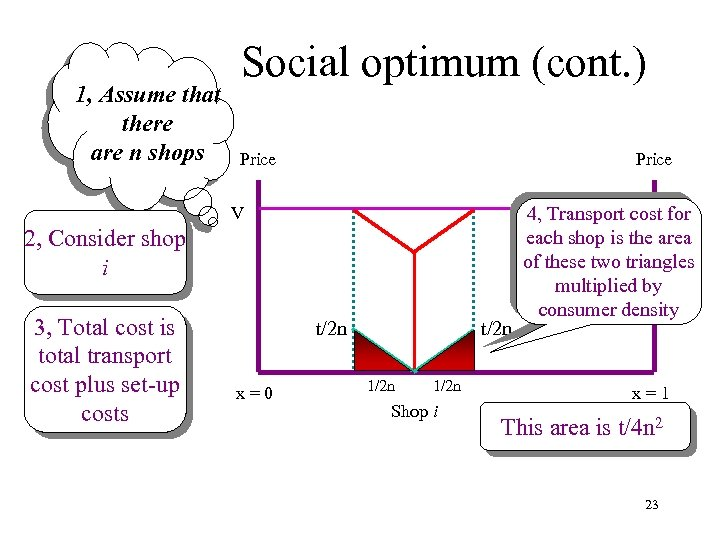1, Assume that there are n shops Social optimum (cont. ) Price V 2, Consider shop i 3, Total cost is total transport cost plus set-up costs t/2 n x=0 t/2 n 1/2 n Shop i V 4, Transport cost for each shop is the area of these two triangles multiplied by consumer density x=1 This area is t/4 n 2 23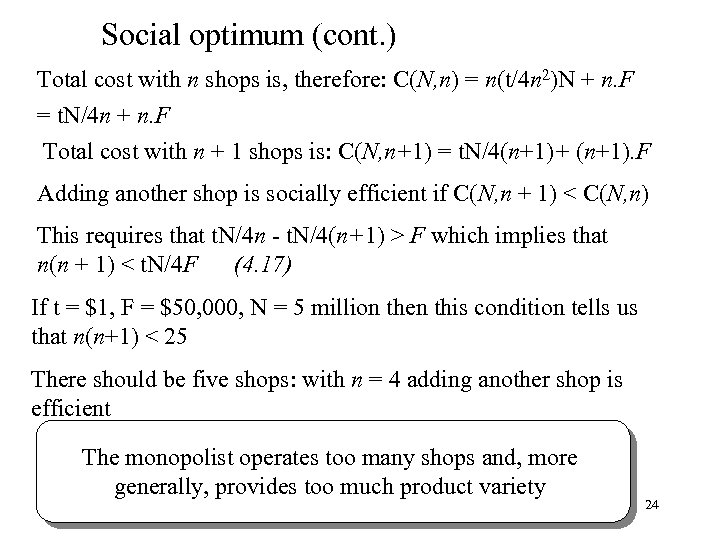Social optimum (cont. ) Total cost with n shops is, therefore: C(N, n) = n(t/4 n 2)N + n. F = t. N/4 n + n. F Total cost with n + 1 shops is: C(N, n+1) = t. N/4(n+1)+ (n+1). F Adding another shop is socially efficient if C(N, n + 1) < C(N, n) This requires that t. N/4 n - t. N/4(n+1) > F which implies that n(n + 1) < t. N/4 F (4. 17) If t = \$1, F = \$50, 000, N = 5 million then this condition tells us that n(n+1) < 25 There should be five shops: with n = 4 adding another shop is efficient The monopolist operates too many shops and, more generally, provides too much product variety 24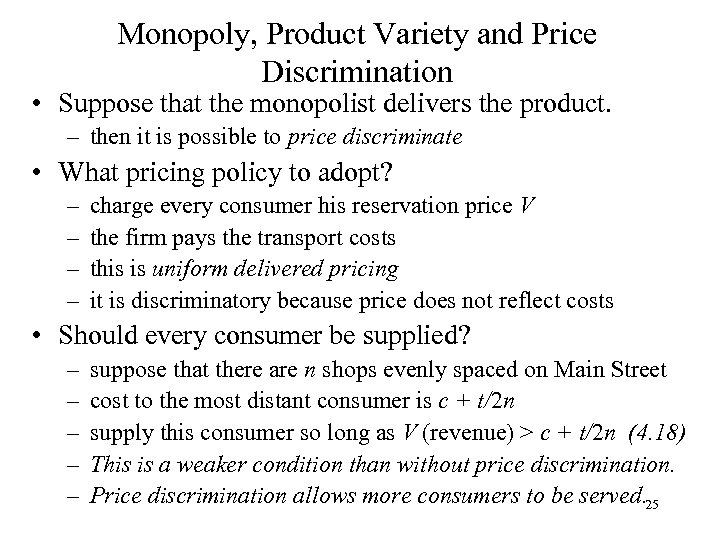Monopoly, Product Variety and Price Discrimination • Suppose that the monopolist delivers the product. – then it is possible to price discriminate • What pricing policy to adopt? – – charge every consumer his reservation price V the firm pays the transport costs this is uniform delivered pricing it is discriminatory because price does not reflect costs • Should every consumer be supplied? – – – suppose that there are n shops evenly spaced on Main Street cost to the most distant consumer is c + t/2 n supply this consumer so long as V (revenue) > c + t/2 n (4. 18) This is a weaker condition than without price discrimination. Price discrimination allows more consumers to be served. 25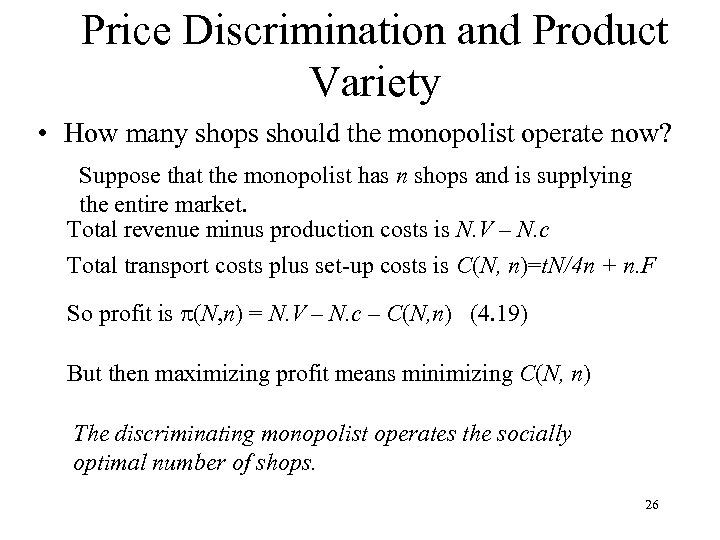Price Discrimination and Product Variety • How many shops should the monopolist operate now? Suppose that the monopolist has n shops and is supplying the entire market. Total revenue minus production costs is N. V – N. c Total transport costs plus set-up costs is C(N, n)=t. N/4 n + n. F So profit is p(N, n) = N. V – N. c – C(N, n) (4. 19) But then maximizing profit means minimizing C(N, n) The discriminating monopolist operates the socially optimal number of shops. 26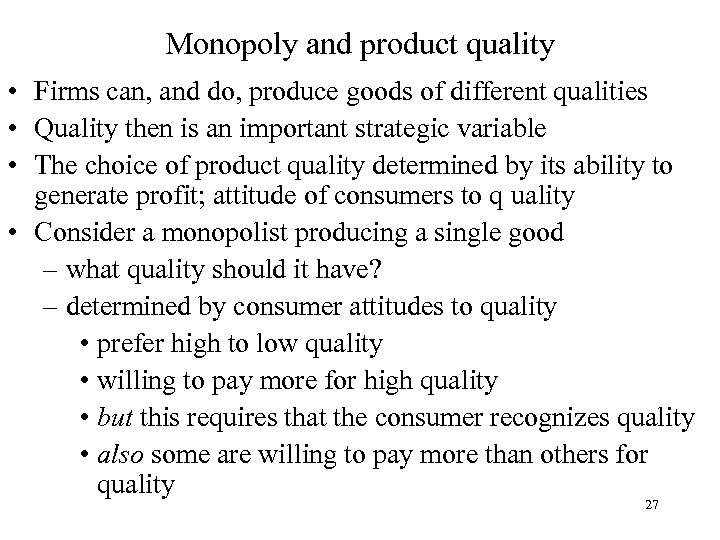Monopoly and product quality • Firms can, and do, produce goods of different qualities • Quality then is an important strategic variable • The choice of product quality determined by its ability to generate profit; attitude of consumers to q uality • Consider a monopolist producing a single good – what quality should it have? – determined by consumer attitudes to quality • prefer high to low quality • willing to pay more for high quality • but this requires that the consumer recognizes quality • also some are willing to pay more than others for quality 27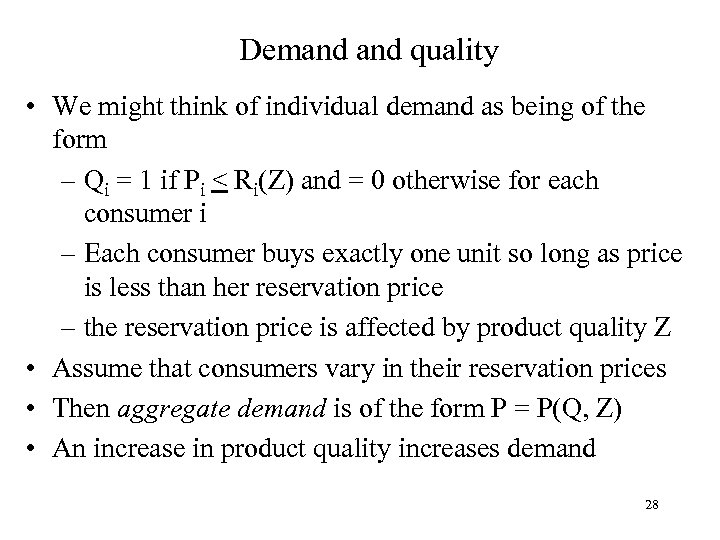Demand quality • We might think of individual demand as being of the form – Qi = 1 if Pi < Ri(Z) and = 0 otherwise for each consumer i – Each consumer buys exactly one unit so long as price is less than her reservation price – the reservation price is affected by product quality Z • Assume that consumers vary in their reservation prices • Then aggregate demand is of the form P = P(Q, Z) • An increase in product quality increases demand 28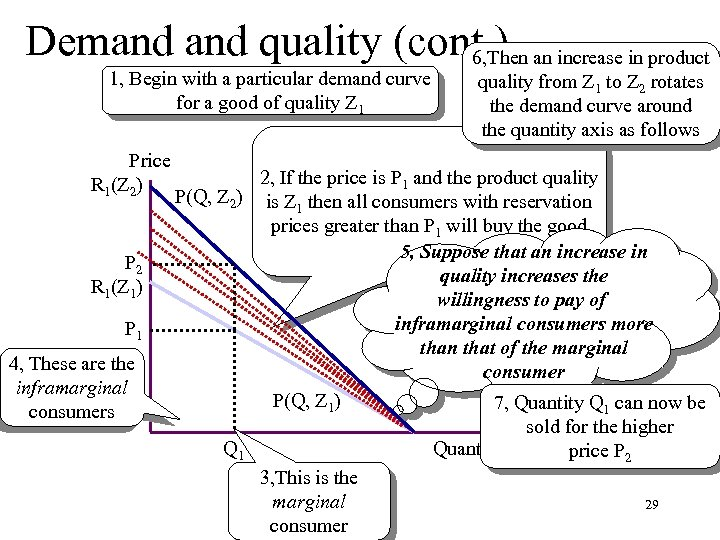Demand quality (cont. ) an increase in product 6, Then 1, Begin with a particular demand curve for a good of quality Z 1 Price R 1(Z 2) P 2 R 1(Z 1) P 1 4, These are the inframarginal consumers quality from Z 1 to Z 2 rotates the demand curve around the quantity axis as follows 2, If the price is P 1 and the product quality P(Q, Z 2) is Z then all consumers with reservation 1 prices greater than P 1 will buy the good 5, Suppose that an increase in quality increases the willingness to pay of inframarginal consumers more than that of the marginal consumer P(Q, Z 1) 7, Quantity Q 1 can now be sold for the higher Q 1 Quantity price P 2 3, This is the marginal 29 consumer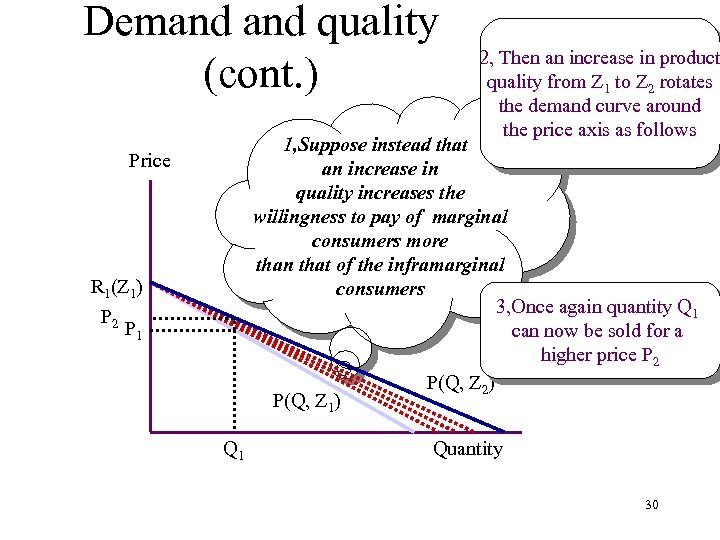Demand quality (cont. ) 2, Then an increase in product quality from Z 1 to Z 2 rotates the demand curve around the price axis as follows 1, Suppose instead that an increase in quality increases the willingness to pay of marginal consumers more than that of the inframarginal consumers 3, Once again quantity Q 1 can now be sold for a higher price P 2 P(Q, Z 2) P(Q, Z 1) Price R 1(Z 1) P 2 P 1 Quantity 30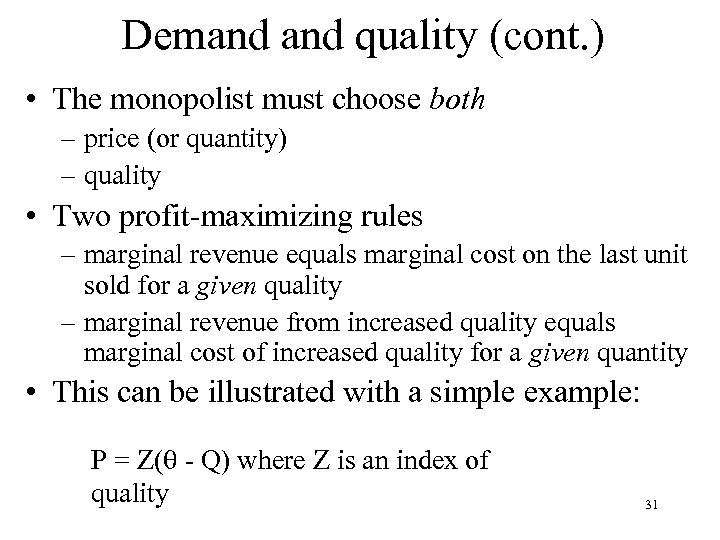Demand quality (cont. ) • The monopolist must choose both – price (or quantity) – quality • Two profit-maximizing rules – marginal revenue equals marginal cost on the last unit sold for a given quality – marginal revenue from increased quality equals marginal cost of increased quality for a given quantity • This can be illustrated with a simple example: P = Z( - Q) where Z is an index of quality 31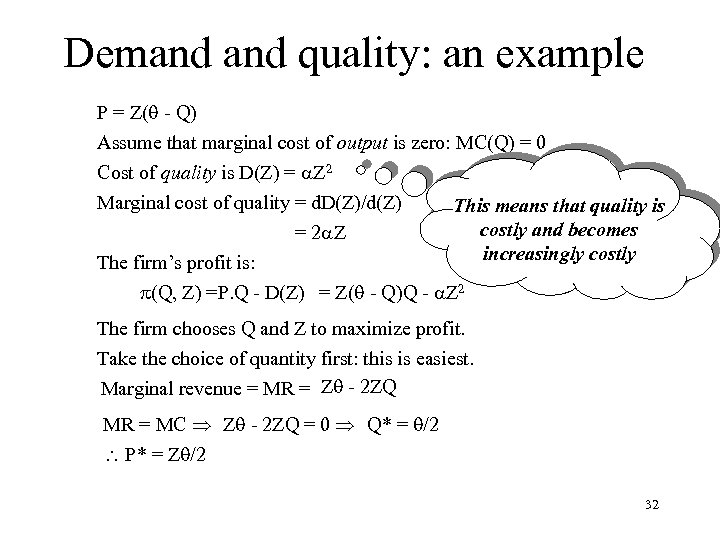Demand quality: an example P = Z( - Q) Assume that marginal cost of output is zero: MC(Q) = 0 Cost of quality is D(Z) = a. Z 2 Marginal cost of quality = d. D(Z)/d(Z) This means that quality is costly and becomes = 2 a. Z increasingly costly The firm’s profit is: p(Q, Z) =P. Q - D(Z) = Z( - Q)Q - a. Z 2 The firm chooses Q and Z to maximize profit. Take the choice of quantity first: this is easiest. Marginal revenue = MR = Z - 2 ZQ MR = MC Z - 2 ZQ = 0 Q* = /2 P* = Z /2 32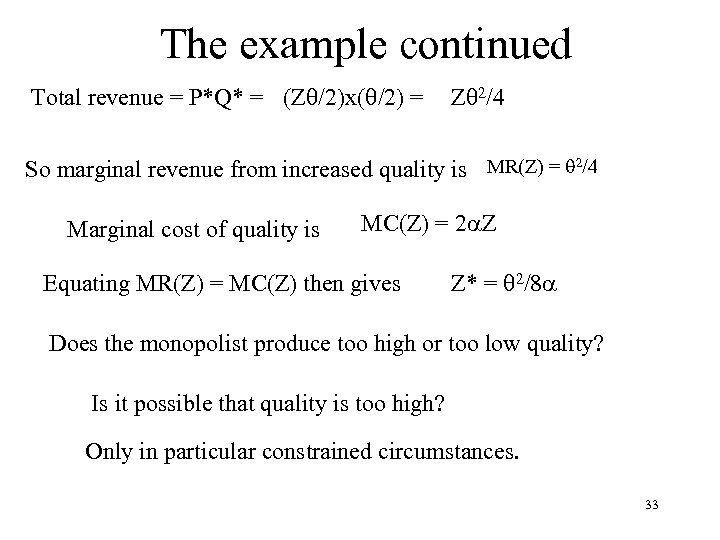The example continued Total revenue = P*Q* = (Z /2)x( /2) = Z 2/4 So marginal revenue from increased quality is MR(Z) = 2/4 Marginal cost of quality is MC(Z) = 2 a. Z Equating MR(Z) = MC(Z) then gives Z* = 2/8 a Does the monopolist produce too high or too low quality? Is it possible that quality is too high? Only in particular constrained circumstances. 33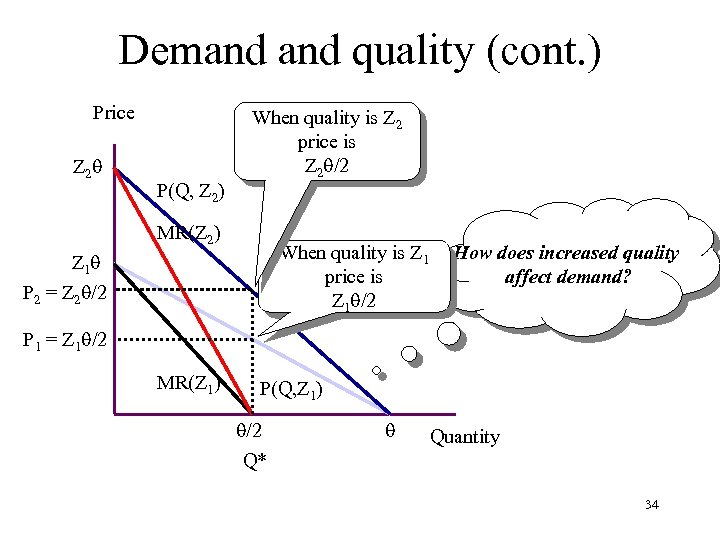Demand quality (cont. ) Price Z 2 P(Q, Z 2) When quality is Z 2 price is Z 2 /2 MR(Z 2) When quality is Z 1 price is Z 1 /2 Z 1 P 2 = Z 2 /2 How does increased quality affect demand? P 1 = Z 1 /2 MR(Z 1) P(Q, Z 1) /2 Q* Quantity 34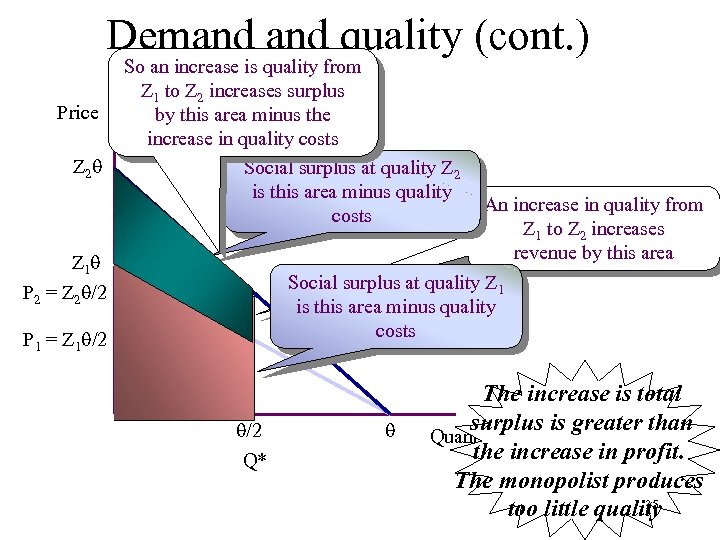Demand quality (cont. ) Price Z 2 So an increase is quality from Z 1 to Z 2 increases surplus by this area minus the increase in quality costs Social surplus at quality Z 2 is this area minus quality costs Z 1 An increase in quality from Z 1 to Z 2 increases revenue by this area Social surplus at quality Z 1 is this area minus quality costs P 2 = Z 2 /2 P 1 = Z 1 /2 Q* The increase is total surplus is greater than Quantity the increase in profit. The monopolist produces 35 too little quality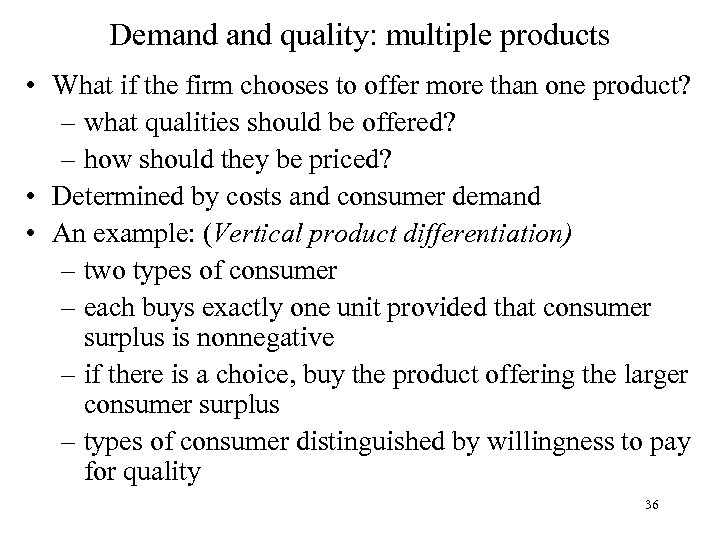Demand quality: multiple products • What if the firm chooses to offer more than one product? – what qualities should be offered? – how should they be priced? • Determined by costs and consumer demand • An example: (Vertical product differentiation) – two types of consumer – each buys exactly one unit provided that consumer surplus is nonnegative – if there is a choice, buy the product offering the larger consumer surplus – types of consumer distinguished by willingness to pay for quality 36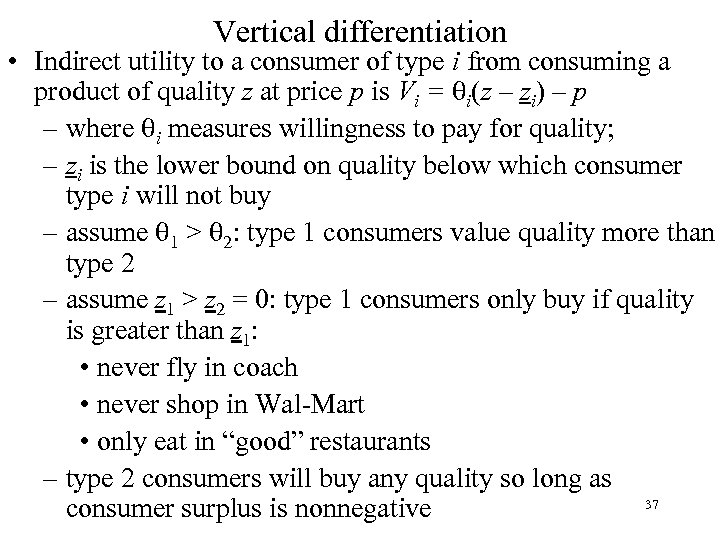Vertical differentiation • Indirect utility to a consumer of type i from consuming a product of quality z at price p is Vi = i(z – zi) – p – where i measures willingness to pay for quality; – zi is the lower bound on quality below which consumer type i will not buy – assume 1 > 2: type 1 consumers value quality more than type 2 – assume z 1 > z 2 = 0: type 1 consumers only buy if quality is greater than z 1: • never fly in coach • never shop in Wal-Mart • only eat in “good” restaurants – type 2 consumers will buy any quality so long as 37 consumer surplus is nonnegative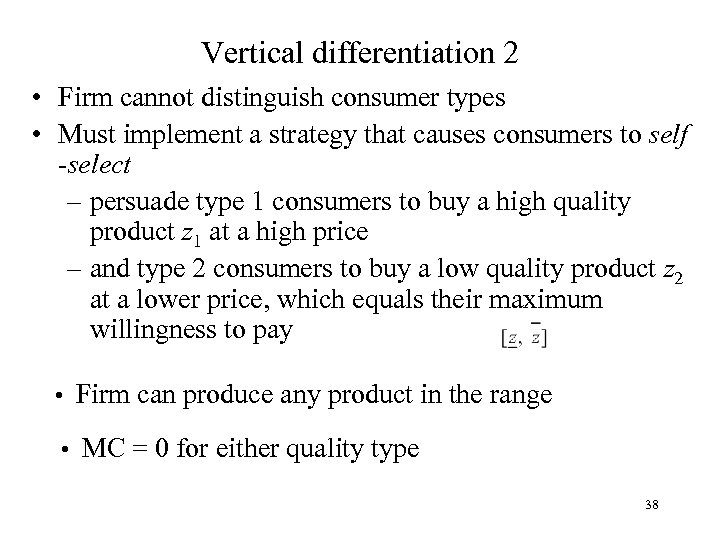Vertical differentiation 2 • Firm cannot distinguish consumer types • Must implement a strategy that causes consumers to self -select – persuade type 1 consumers to buy a high quality product z 1 at a high price – and type 2 consumers to buy a low quality product z 2 at a lower price, which equals their maximum willingness to pay • Firm can produce any product in the range • MC = 0 for either quality type 38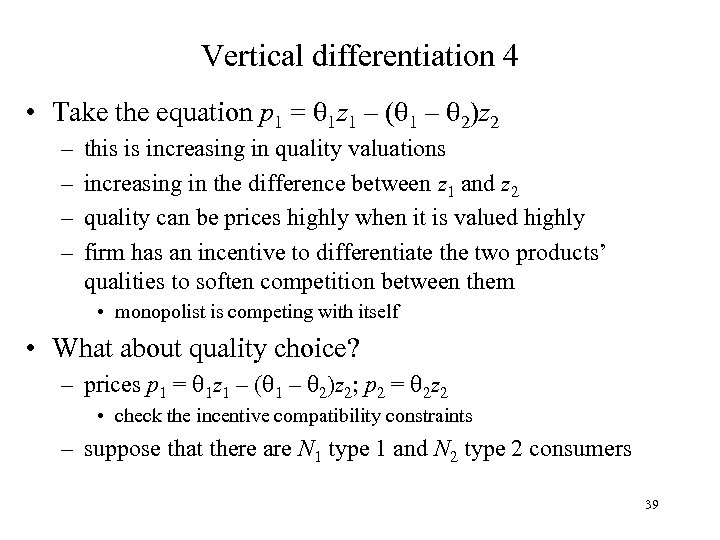Vertical differentiation 4 • Take the equation p 1 = 1 z 1 – ( 1 – 2)z 2 – – this is increasing in quality valuations increasing in the difference between z 1 and z 2 quality can be prices highly when it is valued highly firm has an incentive to differentiate the two products’ qualities to soften competition between them • monopolist is competing with itself • What about quality choice? – prices p 1 = 1 z 1 – ( 1 – 2)z 2; p 2 = 2 z 2 • check the incentive compatibility constraints – suppose that there are N 1 type 1 and N 2 type 2 consumers 39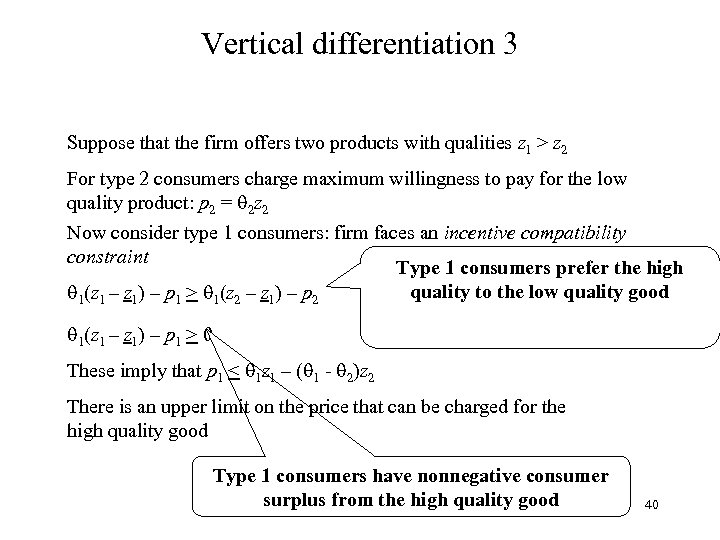Vertical differentiation 3 Suppose that the firm offers two products with qualities z 1 > z 2 For type 2 consumers charge maximum willingness to pay for the low quality product: p 2 = 2 z 2 Now consider type 1 consumers: firm faces an incentive compatibility constraint Type 1 consumers prefer the high quality to the low quality good 1(z 1 – z 1) – p 1 > 1(z 2 – z 1) – p 2 1(z 1 – z 1) – p 1 > 0 These imply that p 1 < 1 z 1 – ( 1 - 2)z 2 There is an upper limit on the price that can be charged for the high quality good Type 1 consumers have nonnegative consumer surplus from the high quality good 40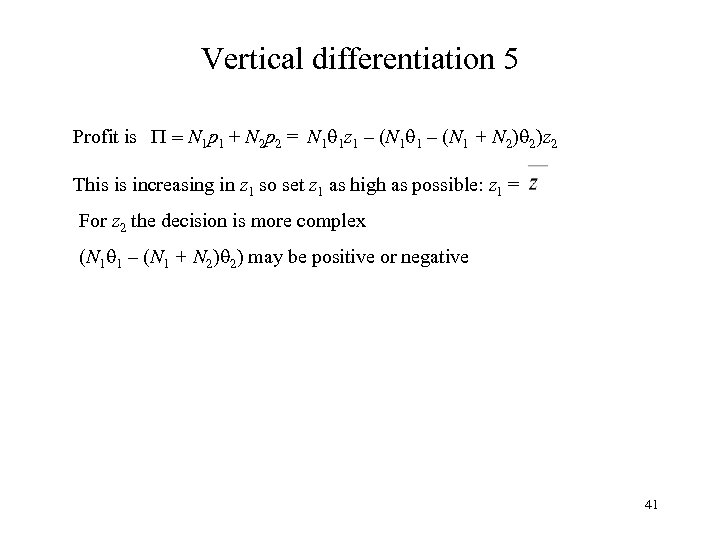Vertical differentiation 5 Profit is P = N 1 p 1 + N 2 p 2 = N 1 1 z 1 – (N 1 + N 2) 2)z 2 This is increasing in z 1 so set z 1 as high as possible: z 1 = For z 2 the decision is more complex (N 1 1 – (N 1 + N 2) 2) may be positive or negative 41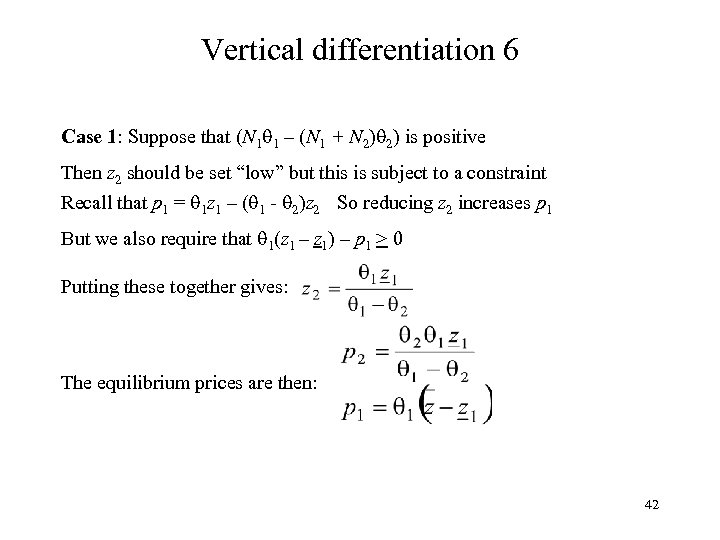Vertical differentiation 6 Case 1: Suppose that (N 1 1 – (N 1 + N 2) 2) is positive Then z 2 should be set “low” but this is subject to a constraint Recall that p 1 = 1 z 1 – ( 1 - 2)z 2 So reducing z 2 increases p 1 But we also require that 1(z 1 – z 1) – p 1 > 0 Putting these together gives: The equilibrium prices are then: 42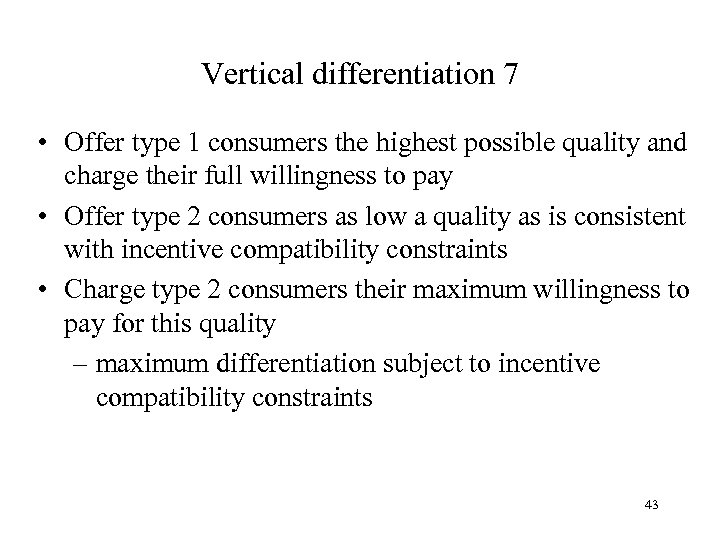Vertical differentiation 7 • Offer type 1 consumers the highest possible quality and charge their full willingness to pay • Offer type 2 consumers as low a quality as is consistent with incentive compatibility constraints • Charge type 2 consumers their maximum willingness to pay for this quality – maximum differentiation subject to incentive compatibility constraints 43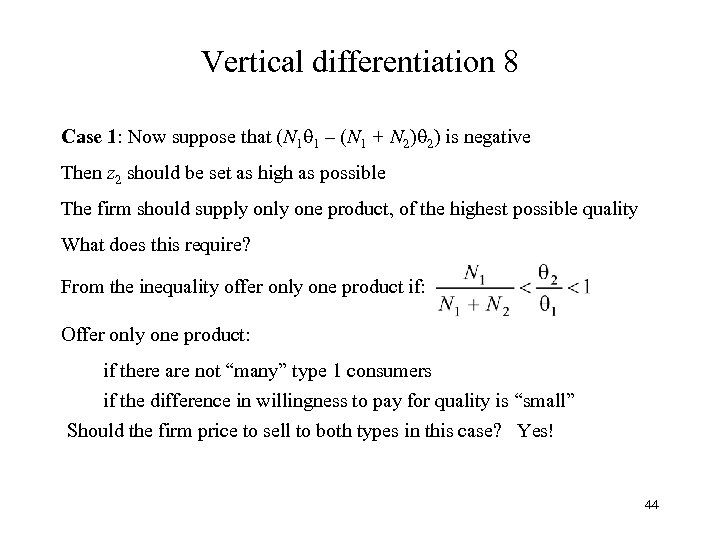Vertical differentiation 8 Case 1: Now suppose that (N 1 1 – (N 1 + N 2) 2) is negative Then z 2 should be set as high as possible The firm should supply one product, of the highest possible quality What does this require? From the inequality offer only one product if: Offer only one product: if there are not “many” type 1 consumers if the difference in willingness to pay for quality is “small” Should the firm price to sell to both types in this case? Yes! 44